Reach Us+44-1474-556909
Magnetohydrodynamic (MHD) Stability of Oscillating Fluid Cylinder with Magnetic Field | OMICS International
Journal of Applied & Computational Mathematics
All submissions of the EM system will be redirected to Online Manuscript Submission System. Authors are requested to submit articles directly to Online Manuscript Submission System of respective journal.

# Magnetohydrodynamic (MHD) Stability of Oscillating Fluid Cylinder with Magnetic Field

Barakat HM*

Department of Mathematics, Faculty of Science and arts, Al Jouf University, Al Jouf, The Kingdom of Saudi Arabia

*Corresponding Author:
Barakat HM
Department of Mathematics
Faculty of Science and arts
Al Jouf University
Al Jouf, The Kingdom of Saudi Arabia
Tel:+96646257869
E-mail: [email protected]

Received: November 09, 2015; Accepted: December 09, 2015; Published: December 14, 2015

Citation: Barakat HM (2015) Magnetohydrodynamic (MHD) Stability of Oscillating Fluid Cylinder with Magnetic Field. J Appl Computat Math 4:271. doi:10.4172/2168-9679.1000271

Copyright: © 2015 Barakat HM. This is an open-access article distributed under the terms of the Creative Commons Attribution License, which permits unrestricted use, distribution, and reproduction in any medium, provided the original author and source are credited.

Visit for more related articles at Journal of Applied & Computational Mathematics

#### Abstract

The magnetohydrodynamic (MHD) stability of oscillating fluid with longitudinal magnetic field has been discussed. The problem is formulated and the (MHD) basic equations are solved. By using the computer procedure from different values of the acting magnetic field the stable and unstable regions are identified. This phenomenon is interest, academically and during the geological drilling in the crust of the earth as we have superposed gas-oil layer mixture fluids. A general eigenvalue relation is derived studied analytically and results are confirmed numerically. The oscillating liquid has stabilizing tendency, in the absence of the effect of the electromagnetic field in the liquid and gas cylinder region, so the model is only subject to the capillary force. It has been found that the model is unstable in the region 0 < x < 1, while it is stable in the region 1 ≤ x < ∞ where x is the longitudinal dimensionless wave number. This means that the model is just unstable in small domains of axisymmetric perturbation but it stables in all domains. For very high intensity of magnetic field the model is completely stable for all values of wavelengths. The capillary force is destabilizing only in a small axisymmetric domain while it is stabilizing in all other axisymmetric perturbations. The stability behavior of the model comes after destabilizing behavior of the model when it be reduced and suppressed.

#### Keywords

Magnetohydrodynamic; Magnetic field; Electromagnetic field; Fluid cylinder

#### Introduction

The classical of the capillary instability of a gas cylinder submerged into a liquid are given for first time by Chandrasekhar for axisymmetric perturbation. Hasan , Elazab et al.  and Drazin and Reid  gave the dispersion relation valid for all axisymmetric and non- axisymmetric modes. Cheng  discussed the instability of a gas jet in an incompressible liquid for all modes of perturbation. However, we have to mention here that the results given by Cheng . Kindall  performed experiments with modern equipment to check the breaking up of that model. Moreover, he attracted the attention for the importance of the stability and discussions of that model for its application in many domains of science. Concerning the hydrodynamic stability of a hollow jet endowed with surface tension we may refer to Chauhan et al., Abramowitz and Stegun , Chen and Lin , Cousin and Dumouchel , Lee and Wang [11,12], Mehring and Sirignano , Parthasarathy and Chiang , Shen and Li , Shi et al. , Shukudov and Sisoev , and Villermaux , Melachlan and Kelly [19,20]. Soon afterwards a lot of researchers treated with the magneto-dynamic stability of such model analytically [21-23] and numerically upon utilizing appropriate basic equation and boundary condition. Hamdy M Brakat and Khloud R Khater studied the Hydromagnetic self-gravitating stability of streaming fluid cylinder with longitudinal magnetic filed . It all foregoing works the liquid may be at rest or unform streaming in the unperturbed state. Here we explain the hydromagnetic stability of oscillating fluid cylinder with longitudinal magnetic field.

Formulation of the problem

We consider a fluid cylinder (with negligible motion) of radius R0 surrounded by an oscillating liquid cylinder with velocity,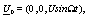(1)

Where U and Ω are the amplitude and oscillation frequency of the velocity.

The interior cylinder is being a gas with constant pressure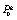and pervaded by the longitudinal magnetic field,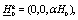(2)

and the liquid is penetrated by magnetic field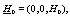(3)

where H0 is the intensity of the magnetic field and α is the parameter of H0g. The components of equations (1 - 3) are considered along the cylindrical polar coordinates (r, Φ, z) with the z-axis is coinciding with the axis of the hollow jet. The model is acted by the inertia, pressure gradient, capillary and electromagnetic forces. The hydro-magnetic fundamental equations appropriate for studying the stability of the fluid model under consideration are the combination of the pure hydrodynamic equations and those of Maxwell concerning the electromagnetic theory.

These equations may be given as follows in the liquid region.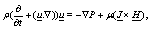(4)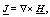(5)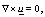(6)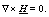(7)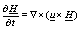(8)

In the region surrounding the gas cylinder, we have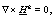(9)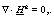(10)

and along the gas- liquid interface the curvature pressure is,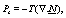(11)

where H and Hg are the magnetic field intensities in the gas and liquid regions, ρ is the mass density, u velocity vector, P kinetic pressure, and Ps the curvature pressure due to the capillary force, T the surface tension coefficient, N is the unite outward normal vector to the gasliquid interface. In the unperturbed state equation (4) reduces to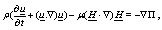(12)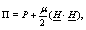(13)

where Π is the total hydromagnetics pressure which is the sum of the liquid kinetic pressure and magnetic pressure, and equation (8) can be rewritten as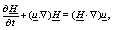(14)

using the equations (4) and (14) we get,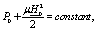(15)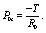(16)

The continuity of the total stress tensor across the gas-liquid interface at (r = R0) yields, the unperturbed pressure P0 of the liquid as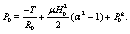(17)

Here P0g is the gas constant pressure in the initial state,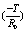is the contribution of capillary force, while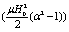is the net magnetic pressure due to the effect of electromagnetic force acting in the gas and liquid regions,

Perturbation analysis

For small departures from the equilibrium state due to an infinitesimal perturbation every perturbed quantity Q(r, q, z, t) can be expanded as;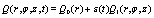(18)

where the quantities with subscript 0 are those of equilibrium while those with 1 are small increments due to the perturbation. The Q(r, ?, z, t) stands for H,H(g) , p and U, Ps and N and the perturbed radial distance of the fluid cylinder.

The amplitude ε of the perturbation is given by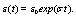(19)

Where ε0 is the initial amplitude at (t=0) and σ is the temporal amplification of instability. Consider a sinusoidal wave along the gasliquid interface, using a single fourier term, the perturbed cylinder radial distance of the gas jet is described by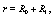(20)

where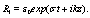(21)

Is the elevation of the surface wave measured from unperturbed position. Here K (any real number) is the longitudinal wave number, and m an integer is the azimuthal wave number. In view of the foregoing expansions (18 - 21), the relevant perturbation equations are given as follows. For the interior of the cylindrical jet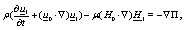(22)

where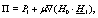(23)

and by using equations (6), (7) and (8) we get,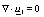(24)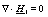(25)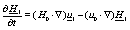(26)

In the gas region,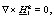(27)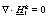(28)

as along the gas-liquid interface,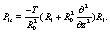(29)

Based on the equations (18 - 21) every fluctuating quantity Q1(r, 0, z, t) could be written as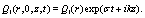(30)

By the aid of this expansion, equations (22) and (24) are combined to give magnetic field intensity in the form,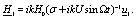(31)

Since the liquid is assumed to be non-dissipative and irrotational, the velocity u1could be derived from a scalar function Φ1(r, 0, z, t) such that,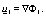(32)

Combining equations (24) and (32) we get,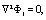(33)

by using equation (28) means the magnetic field H1g could bederive from a scalar functions sayΨ1g , such that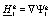(34)

Combining equations (28) and (34) we get,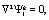(35)

by using equations (22) and (31) we find,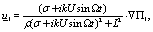(36)

where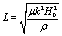. So by using equations (24) we get,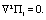(37)

Now,we may see that the system of perturbed equations (22) and (24 -28) could be solved, as Laplace,s equations (33), (35) and (37) are solved for this task, we may write the expansion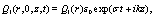(38)

for Φ1(r, 0, z, t), Ψ1(r, 0, z, t) and Π1(r, 0, z, t). Substituting equation (38) into equations (33), (35) and (37), we obtain the total second order differential equation of Bessel. For the problem under consideration, the nonsingular solutions of (33), (35) and (37),are given by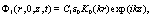(39)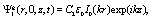(40)(41)

Where C1,C2 and C3 are constant of integration to be determined, while K0 and I0 are Bessel functions of the first and second kind order m = 0. Along the gas-liquid cylinder fluid interface, the surface pressure in the perturbed state due to the capillary force, in view of equations (22) and (29), given by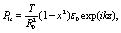(42)

Where (x = kR0) is the longitudinal dimensionless wave number.

#### Boundary Condition

The solution of the unperturbed and perturbed sates equations (39),(41) must satisfy appropriate boundary condition across the fluid interface at (r = R0), these conditions are given as follows

Kinematic condition

The first condition states that "the normal component of the velocity u of the liquid must be compatible with the perturbed boundary gas liquid at (r = R0). This condition is given,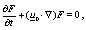(43)

so we can get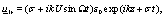(44)

by using equation (39) and (32) we have,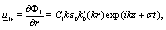(45)

then,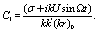(46)

The second boundary condition could be described and utilized as follows, by using equations (24) and (31) we have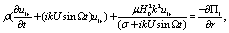(47)

where,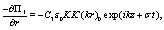(48)

by using equation(48) we obtain,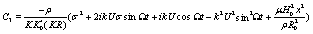(49)

The magneto-dynamic condition

This condition is being "the normal component of the magnetic field must be continuous across the gas-liquid interface at (r = R0)",mathematically this condition is given by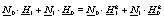(50)

with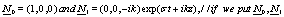in equation (50) we have,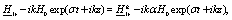(51)

where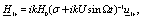(52)

by using equations (46), (40) and (34) we get,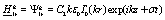(53)

substituting in equation (51) we get the value of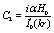(54)

Stresses condition

This condition is being" The jump of the normal component of the stresses in the gas and liquid regions must be discontinuous by the surface pressure P1s across the cylindrical gas-liquid interface at (r = R0) ". This condition is being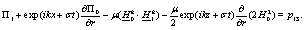(55)

From this equation we get the dispersion relation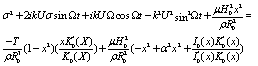(56)

#### General Discussion

The relation (56) is the dispersion equation of a fluid cylinder acting upon the electromagnetic force. It relates the growth rate σ with the modified Bessel function I0(x) and K0(x) and their derivative, the wave number m and x, the amplitude U of the streaming velocity, Ω the oscillation frequency of the oscillating streaming, α the parameter of the magnetic field in the gas cylinder and with the parameters T, ρ, R0, μ and H0 of the problem. One has to mention here that the relation (56) contains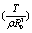as well as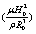as unit of (time)2 and this fact is vital in formulating (56) in dimensionless form. Relation (56) is a simple linear combination of eigenvalue relations of gas-liquid cylinder subject to the electromagnetic force only.It is remarkable that in the axisymmetric mode at m = 0 for a fluid cylindrical jet pervaded by constant axial field, subject to its own attraction and lorentz force. This character of a simple linear combination is also true if the acting force are the capillary and electromagnetic forces. Whether the model are a full liquid-gas cylinder. The physical interpretation of such phenomenon is given explicitly in this paper reference. Since the relation (56) is somewhat more general, several stability criteria can be obtained as limiting cases from the eigenvalue of relation (60). The discussion of this equation reveals that the uniform streaming of the liquid has destabilizing effect, and that effect is valid not only in the axisymmetric mode m = 0.

If we assume that U = 0, then the eigenvalues relation(56) will be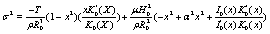(57)

In order to examine the effects of the capillary and magneto dynamic forces on the stability of the present model, we have to write down about some properties of the modified Bessel functions, consider the recurrence relation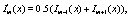(58)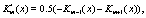(59)

by using the Wronskian relation,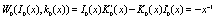(60)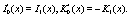(61)

Then the dispersion relation will be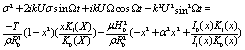(62)

so, at U = 0, Ω = 0, H0 = 0, the relation (62) reduces to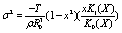(63)

This relation has been given by Drazin and Reid , which is valid for all axisymmetric and non-axismmetric modes (m = 0, m ≥ 1) of perturbation.

The discussion of the dispersion relation (63) reveals that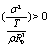as (0 < x < 1) when (m = 0)

This,means that the hollow gas jet is capillary unstable only in the axisymmetric mode (m = 0) in the small domain (0 < x < 1).

As at (U = 0, T0 = 0, and Ω = 0) we have from equation (62)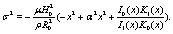(64)

The axial magnetic field pervaded in the liquid is represented by the term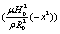. It has strong stabilizing effect and that effect is independent of the perturbed modes (m = 0) and (m = 1). The effect of the magnetic field pervaded in the gas cylinder is represented by the term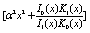followed by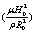. It has strong stabilizing effect and this effect is valid for all axisymmetric mode m = 0 and non axisymmetric modes m = 1. So we see that the present model is purely stabilizing under the acting electromagnetic forces in gas and liquid regions.

#### Conclusion

From the foregoing discussions, we may conclude the following:

(1) The axial magnetic field pervaded interior the fluid cylinder has stabilizing effect.

(2) The stability behavior of the model comes after the destabilizing behavior of the model when it be reduced and suppressed.

(3) The capillary force is destabilizing only in a small axisymmetric domains and all domains of non-axisymmetric perturbations.

(4) The present model is purely stabilizing under the acting electromagnetic in gas and liquid regions.

(5) For very high intensity of magnetic field the destabilizing character of the model could be suppressed completely for all value of wave lengths.

#### References

Select your language of interest to view the total content in your interested language

### Article Usage

• Total views: 8498
• [From(publication date):
December-2015 - Jul 18, 2019]
• Breakdown by view type
• HTML page views : 8400Can't read the image? click here to refresh